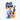# CSAPP Walk Through: Chapter 2

These series of notes are based on the book Computer Systems: A Programmer's Perspective.The homepage for the book is http://csapp.cs.cmu.edu/.

I put these notes here for me to review the book conveniently, hope it helps you as well.

## Imformation Storage

### Bytes

• Byte is the smallest addressable unit of memory.
• Every byte of memory is identified by a unique number(address).
• All possible addresses form the virtual address space.

### Words

• Word size indicates the nominal size of integer and pointer data.
• For a machine with a $w$-bit word size, the virtual memory addresses can range from $0$ to $2^w-1$.

### Data Sizes

This chart shows sizes of C numeric data types of 32-bit and 64-bit machines.

C declaration 32-bit 64-bit
char 1 1
short int 2 2
int 4 4
long int 4 8
long long int 8 8
char * 4 8
float 4 4
double 8 8

### Strings

• A string in C is encoded by an array of characters terminated by the null character(\0).

### Boolean Algebra

• Claude Shannon was the first to made the connection between Boolean algebra and digital logic.
• ** Opeartions: ** NOT, AND, OR and EXCLUSIVE-OR.

### Integer representations

To represent positive-only values(unsigned numbers), we use unsigned encodings. To represent both positive and negative values(signed numbers), we use two's complement encodings.

• U: Unisigned Encodings
• T: Two's Complement Encodings

Remember:

• $U_{Max} = 2^w - 1$
• $T_{Max} = 2^{w-1} - 1$
• $T_{Min} = 2^{w-1}$

### Conversions Between Signed and Unsigend

For conversions between signed and unsigned numbers with the same word size: the numeric values might change, but the bit patterns do not.

When executing an operation between an unsigned operand and a signed operand, C will convert the signed operand to an unsigned operand implicitly.

For conversion from signed integer to unsigned integer:

$$\begin{cases} x+2^w, & x < 0 \\\\ x, & x \ge 0 \end{cases}$$ For conversion from unsigned integer to signed integer: $$\begin{cases} -2^w + u, & u \ge 2^{w-1} \\ u, & u < 2^{w - 1} \end{cases}$$

### Expanding the Bit Representation of a Number

• Zero Extension: To expand a unsigned number, we simply add leading zeros to the representation.
• Sign Extension: To expand a two's complement number, we add copies of the most significant bit to the representation.

### Truncating Numbers

To truncate an unsigned number:

$$B2U_k\left(\left[x_{k-1}, x_{k-2}, ..., x_0\right]\right) = B2U_w\left(\left[x_{w-1}, x_{w-2}, ..., x_0\right]\right) \text{mod} ~ 2^k$$

To truncate an two's complement number:

$$B2T_k\left(\left[x_{k-1}, x_{k-2}, ..., x_0\right]\right) = U2T_k\left(B2U_w\left(\left[x_{w-1}, x_{w-2}, ..., x_0\right]\right) \text{mod} ~ 2^k\right)$$

### Floating Point

The numerical form of floating point numbers is $V_{10} = \left(-1\right)^s \cdot M \cdot 2^E$.

• Sign bit s determines whether the number is positive or negative.
• M is a fractional value in range $\left[1.0, 2.0\right]$
• E weights value by a power of 2.

Floating point in memory:

• Single precision(32 bits): 1 sign bit, 8 exponent bits and 23 fraction bits.
• Double precision(64 bits): 1 sign bit, 11 exponent bits and 52 fraction bits.

exponent bits encodes E, fraction bits encodes M.

Special values:

• When exp bits are all 1 and frac bits are all 0, the number represents $\infty$ ($+\infty$ when $s=0$ and $-\infty$ when $s=1$).
• When exp bits are all 1 and frac bits are not all 0, the number represents $NaN$(Not a Number).

Encoding:

• Exponent was coded as biased values: $E = exp - Bias$.
• Significand coded with implied leading 1: $M = 1.xxx...x$.

Example:

float f = 12345.0;

$12345_{10} = 1.1000000111001_2 \cdot 2^{13}$

Significand:

• $M = 1000000111001_2$.
• $frac = 10000001110010000000000_2$.

Exponent:

• $E = 13$
• $Bias = 127$
• $exp = E + Bias = 140 = 10001100_2$

Result:

sign bit exp frac
0 10001100 10000001110010000000000

# About the author

Hey, I'm Peixuan, a software engineer. I write posts about things I learned.